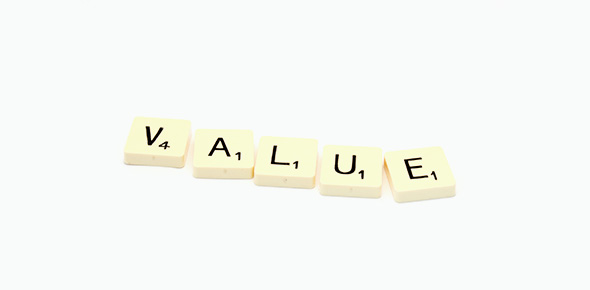# Number And Place Value For Year 2.

8 Questions | Total Attempts: 46SettingsThe following quiz tests the skills of Year 2 students in primary schools for the strand of Number and Algebra. The assessment of each question gives the teacher the evidence of student's understanding the concepts of Numer and Place Value. The quiz reflects assessment of learning against the Australian Curiculum standards and gives foundamental information about some concepts of learning number and place value.

Related Topics
• 1.
Which number is greater than 35?
• A.

34

• B.

35

• C.

36

• D.

10

• 2.
Which words make this statement true? 8 ____ 8
• A.

Is greater than

• B.

Is equal to

• C.

Is less than

• D.

Is more than

• 3.
Is this true or false?  25 is more than 35.
• A.

True

• B.

False

• 4.
Which statement is correct?
• A.

1 hundred means 100 tens.

• B.

28 units is more than 3 tens.

• C.

1 hundred is 10 tens or 100 units.

• D.

5 tens is 47 units.

• 5.
Which sequence shows counting backwards by 5?
• A.

35, 40, 45, 50....

• B.

275, 270, 265, 260....

• C.

95, 85, 75, 65.....

• D.

750, 550, 350, 150

• 6.
How do you write number 458 in words?
• A.

Four hundred fifty eight.

• B.

Four hundreds fifty eight.

• C.

Four hundred five eight.

• D.

Four fifty eight.

• 7.
Does a thousand units equal ten hundreds units?
• A.

True

• B.

False

• 8.
55 is .......... than 35. Choose the correct answer.
• A.

Less

• B.

Greater

• C.

Equal

• D.

Same as